# SN Dey Class 11 Solutions Differentiation Very Short Answer Type Questions

SN Dey Class 11 Differentiation Solutions | Solution of Class 11 Derivative | SN Dey Class 11 Solutions | SN Dey Class 11 Derivative Solutions Very Short Answer Type Questions | West Bengal Board Class 11 Derivative Solution | SN Dey Class 11 Math Chapter Derivative Solution | Differentiation Very Short Answer Type Questions Answers | sn dey class 11 derivatives solutions

## SN Dey Class 11 Differentiation Very Short Answer Type Questions Solutions

SN Dey class 11 Differentiation very short answer type questions Ex 1 solutions:

Ex 1: Define the derivative of a function f(x) at an arbitrary point x in its domain of definition.

Solution:

Let x be a point in the domain of the definition of f(x). The derivative of f(x) at a point x is given by the following limit:

$\lim\limits_{h\to 0}\dfrac{f(x+h)-f(x)}{h}$

and it is denoted by $f'(x)$.

SN Dey class 11 Differentiation very short answer type questions Ex 2 solutions:

Ex 2: Examine whether f(x)=|x| has a derivative at x=0.

Solution:

Let f(x)=|x|. We know that |x|=x if x>0 and |x|=-x if x $\leq$ 0. The left hand derivative of f(x) at x=0 is equal to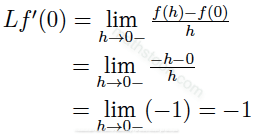And the right hand derivative of f(x) at x=0 is equal to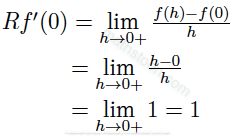As the left hand derivative and the right hand derivative of f(x)=|x| at x=0 are not equal, we conclude that f(x)=|x| has no derivative at x=0. In other words,

$f'(0)$ does not exist.

SN Dey class 11 Differentiation very short answer type questions Ex 3 solutions:

Ex 3: If $y=\frac{1}{x}$, find $\frac{dy}{dx}$; also find the value of x for which dy/dx becomes undefined.

Solution: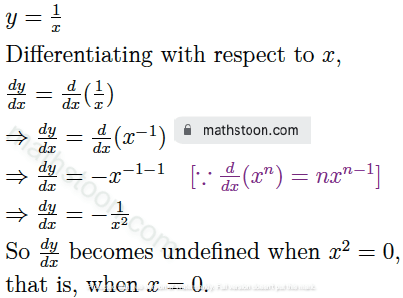SN Dey class 11 Differentiation very short answer type questions Ex 4 solutions: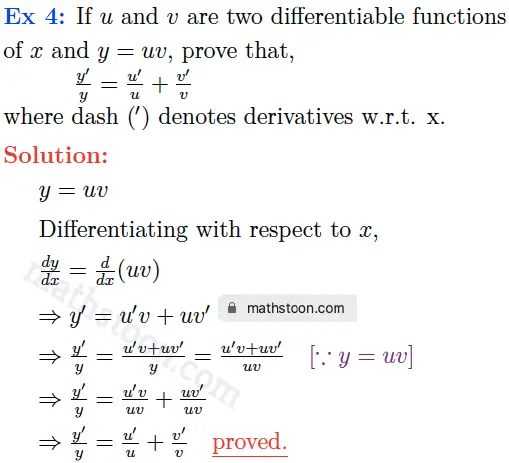SN Dey class 11 Differentiation very short answer type questions Ex 5 solutions: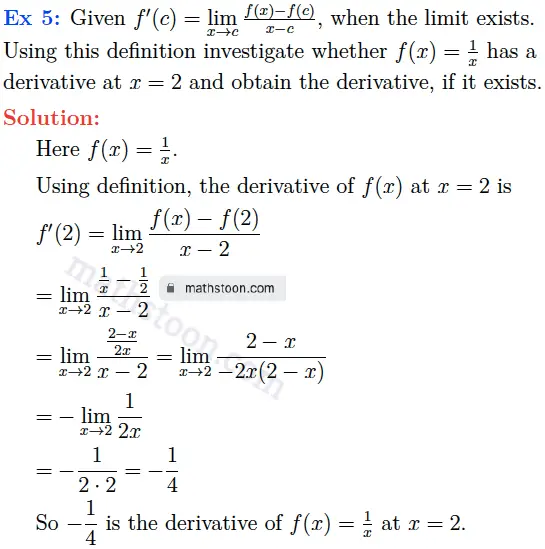Ex 6: Differentiate the following functions w.r.t. x:

Ex 6(i): If y=x16 then find dy/dx.

Solution:

dy/dx= d/dx(x16)

= 16 x16-1 by the power rule of derivatives.

= 16 x15

Ex 6(ii): If y=3x7 then find dy/dx.

Solution:

dy/dx= d/dx(3x7)

= 3 ⋅ 7x7-1 by the power rule of derivatives.

= 21 x6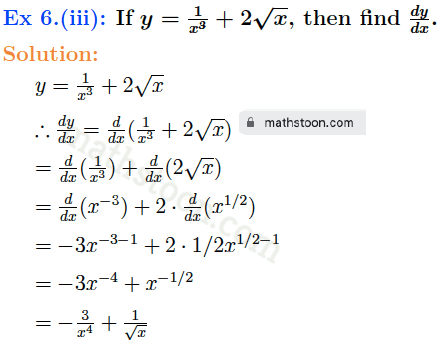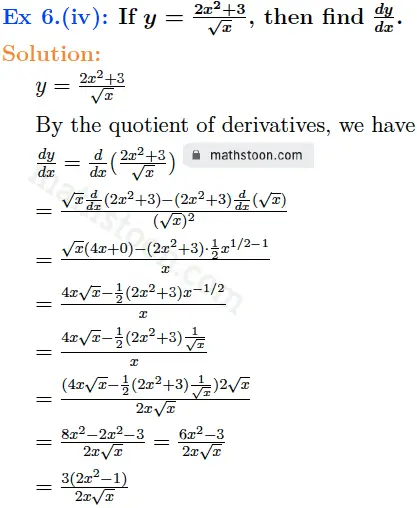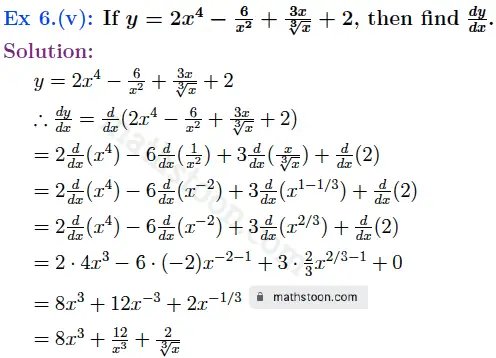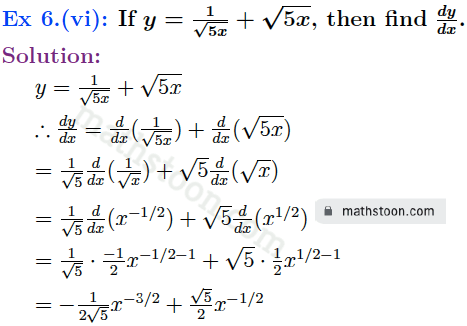Ex 6(vii): If y=(x2-2)2 then find dy/dx.

Solution:

dy/dx= d/dx{(x2-2)2}

= 2(x2 -2) ⋅ d/dx(x2-2)

= 2(x2 -2) ⋅ (2x-0)

= 4x(x2 -2)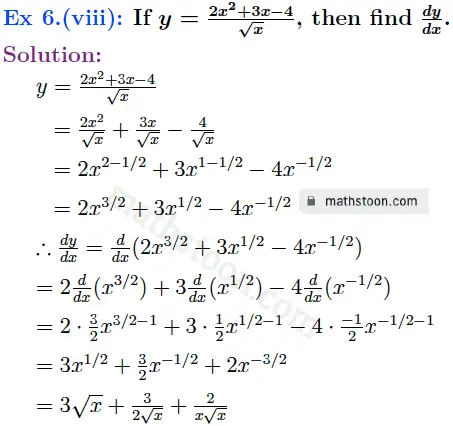Ex 6(ix): If y=(x2-2x)(x+1) then find dy/dx.

Solution:

dy/dx= d/dx{(x2-2x)(x+1)}

= (x2 -2x) d/dx(x+1) + (x+1) d/dx(x2 -2x), by the product rule of derivatives.

= (x2 -2x)(1+0) + (x+1)(2x-2)

= x2 -2x + 2x2 +2x-2x-2

= 3x2 -2x – 2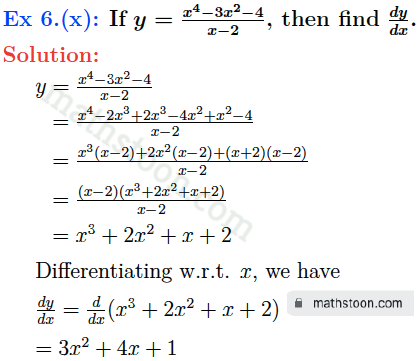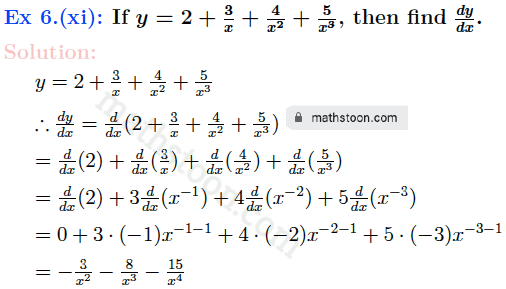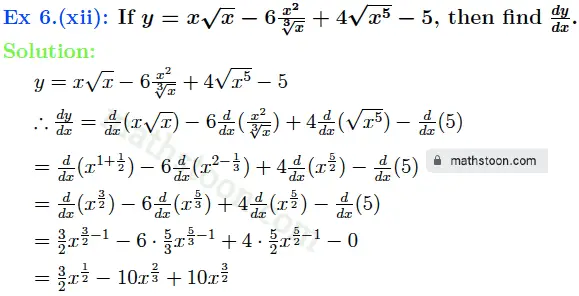Ex 7: Find dy/dx :

Ex 7(i): If y=3log x -4√x+2ex then find dy/dx.

Solution:

y = 3log x -4√x+2ex

∴ dy/dx= d/dx(3log x -4√x+2ex)

= 3 d/dx(log x) – 4 d/dx(√x)+2 d/dx(ex)

= $3 \cdot \frac{1}{x}-$ 4 d/dx(x1/2) + 2ex

= $\frac{3}{x} – 4 \cdot \frac{1}{2}x^{1/2-1}$ + 2ex

= $\frac{3}{x} -2x^{-1/2}$ + 2ex

= $\frac{3}{x} -2x^{-1/2}$ + 2ex

Ex 7(ii): If y = log2 x  then find dy/dx.

Solution:

y = log2

∴ dy/dx= d/dx(log2 x)

= d/dx(log2 e ⋅ loge x)  [∵ logab = logae ⋅logeb]

= log2 e ⋅ d/dx(loge x)

= log2 e ⋅ 1/x =1/x log2e

Ex 7(iii): If y = 2xm-3mx+4ex  then find dy/dx.

Solution:

y = 2xm-3mx+4ex

∴ dy/dx= d/dx(2xm-3mx+4ex)

= 2 ⋅ d/dx(xm) – 3⋅ d/dx(mx)+ 4⋅ d/dx(ex)

= 2 ⋅ mxm-1 – 3⋅ mx logem + 4⋅ ex

= 2mxm-1 – 3mx logem + 4ex

Ex 7(iv): If y = 2x+2 -ex+1 +3log 2x  then find dy/dx.

Solution:

y = 2x+2 -ex+1 +3log 2x

∴ dy/dx= d/dx(2x+2 -ex+1 +3log 2x)

= d/dx(2x+2) – d/dx(ex+1)+ 3⋅ d/dx(log 2x)

= 2x+2 ⋅ loge2 ⋅ d/dx(x+2) – ex+1 ⋅d/dx(x+1) + $3\cdot \frac{1}{2x}\cdot$ d/dx(2x)

= 2x+2 loge2 ⋅ 1 – ex+1 ⋅1 + $3\cdot \frac{1}{2x}\cdot$ 2

= 2x+2 loge2 – ex+1 + $\frac{3}{x}$

Ex 7(v): If y = log10 x +10x +x10+10  then find dy/dx.

Solution:

y = log10 x +10x +x10+10

∴ dy/dx= d/dx(log10 x +10x +x10+10)

= d/dx(log10 x) +d/dx(10x) + d/dx(x10) +d/dx(10)

= $\frac{1}{x}$ log10e + 10xloge10 + 10 x10-1 + 0

= $\frac{1}{x}$ log10e + 10xloge10 + 10x9

Ex 7(vi): If y = ex+1 -5x+1 +elog x+logax +log xa  then find dy/dx.

Solution:

y = ex+1 -5x+1 +elog x+logax +log xa

∴ dy/dx= d/dx(ex+1 -5x+1 +elog x+logax +log xa)

= d/dx(ex+1) -d/dx(5x+1) + d/dx(x) +d/dx(logax) +d/dx(log xa[∵ elog x = x]

= ex+1 d/dx(x+1) – 5x+1 loge5 ⋅ d/dx(x+1) + 1 + $\frac{1}{x}$ logae +axa-1 d/dx(xa

= ex+1(1+0) – 5x+1 loge5 ⋅(1+0) + 1 + $\frac{1}{x}$ logae + $\frac{1}{x^a}$ axa-1

= ex+1 – 5x+1 loge5 + 1 + $\frac{1}{x}$ logae + $\frac{a}{x}$

Ex 7(vii): If y = a sec x + b tan x – c cosec x + d cot x -e  then find dy/dx.

Solution:

y = a sec x + b tan x – c cosec x + d cot x -e

∴ dy/dx= d/dx(a sec x + b tan x – c cosec x + d cot x -e)

= d/dx(a sec x) + d/dx(b tan x) – d/dx(c cosec x) + d/dx(d cot x) – d/dx(e)

= a d/dx(sec x) + b d/dx(tan x) -c d/dx(cosec x) +d d/dx(cot x) – 0

= a sec x tan x + b sec2x +c cosec x cot x -d cosec2x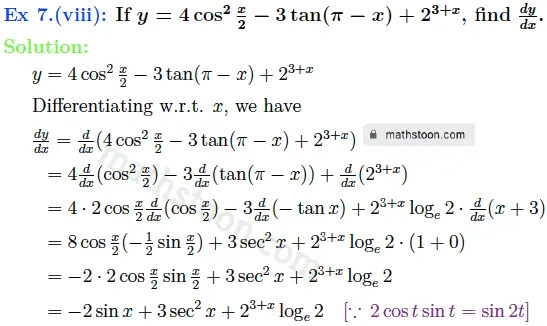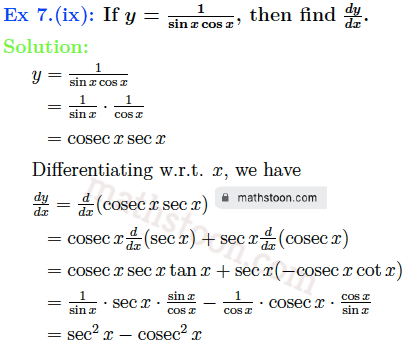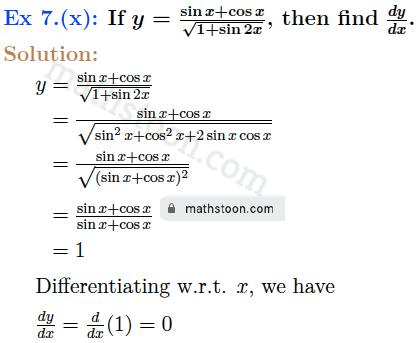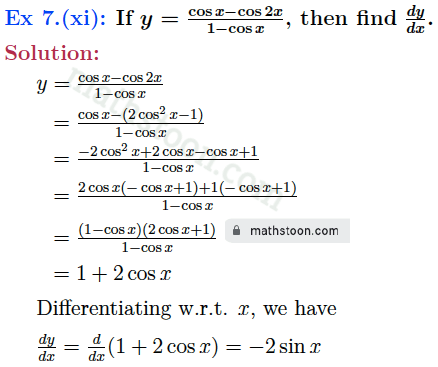SN Dey class 11 Differentiation very short answer type questions Ex 8 solutions:

Ex 8: Find the derivative of cot x by expressing it in the form cos x ⋅ cosec x.

Solution: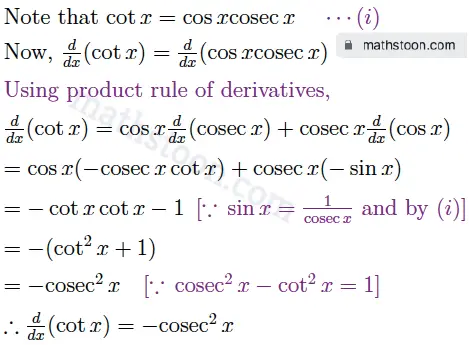SN Dey class 11 Differentiation very short answer type questions Ex 9 solutions: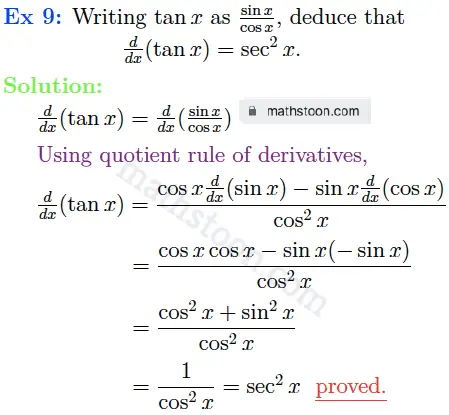SN Dey class 11 Differentiation very short answer type questions Ex 10 solutions: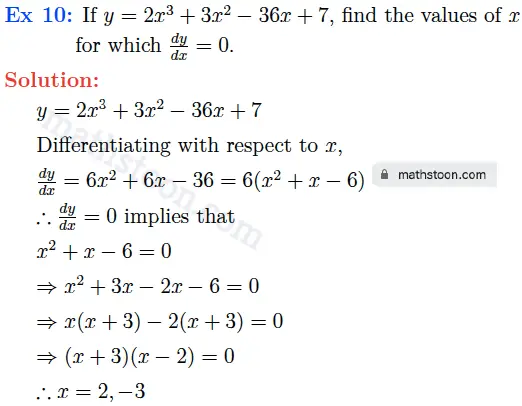SN Dey class 11 Differentiation very short answer type questions Ex 11 solutions: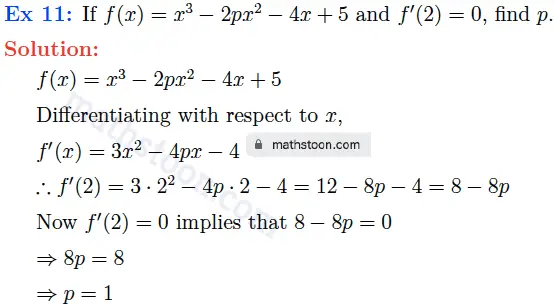SN Dey class 11 Differentiation very short answer type questions Ex 12 solutions: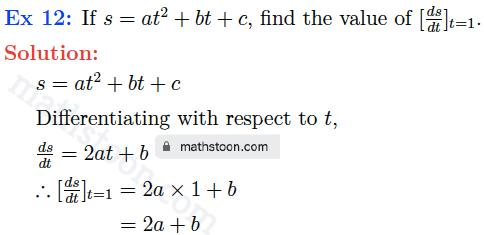SN Dey class 11 Differentiation very short answer type questions Ex 13 solutions: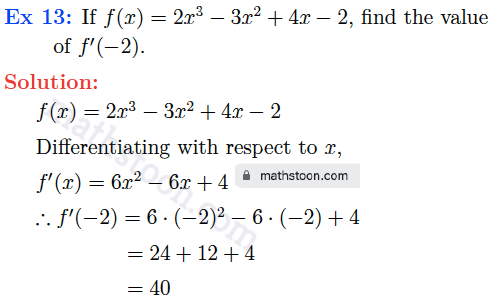SN Dey class 11 Differentiation very short answer type questions Ex 14 solutions:

Ex 14: If $y=x^5$, show that $x \frac{dy}{dx}-5y=0$.

Solution: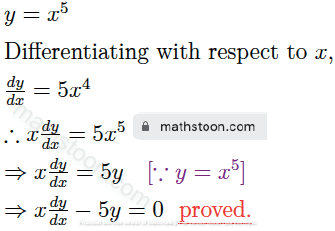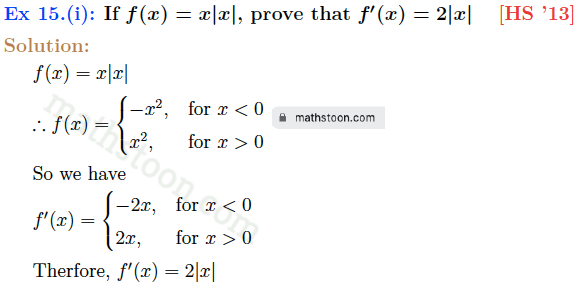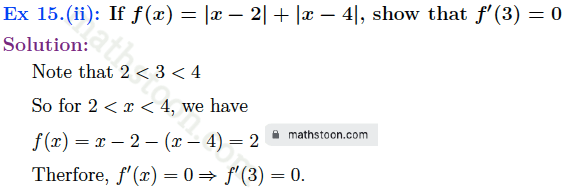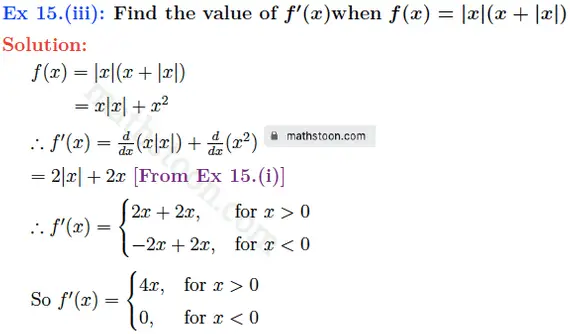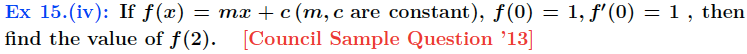Solution:

Given f(x)=mx+c

∴ f(0) = m⋅0+c =c

So f(0)=1 implies that c=1

∴ f(x) = mx+1

Differentiating f(x) with respect to x, we get that

$f'(x) = m$

Now $f'(0)=1$ implies that m=1

Thus, f(x)=x+1

∴ f(2) = 2+1 =3

Share via: